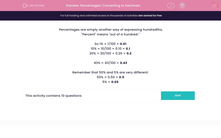# Percentages: Converting to Decimals

In this worksheet, students convert the given percentages into their decimal equivalents.This content is premium and exclusive to EdPlace subscribers.Key stage:  KS 2

Curriculum topic:   Maths and Numerical Reasoning

Curriculum subtopic:   Decimals

Difficulty level:#### Worksheet Overview

Percentages are simply another way of expressing hundredths.

"Percent" means "out of a hundred."

So 1% = 1/100 = 0.01

10% = 10/100 = 0.10 = 0.1

20% = 20/100 = 0.20 = 0.2

43% = 43/100 = 0.43

Remember that 50% and 5% are very different:

50% = 0.50 = 0.5

5% = 0.05

### What is EdPlace?

We're your National Curriculum aligned online education content provider helping each child succeed in English, maths and science from year 1 to GCSE. With an EdPlace account you’ll be able to track and measure progress, helping each child achieve their best. We build confidence and attainment by personalising each child’s learning at a level that suits them.

Get started